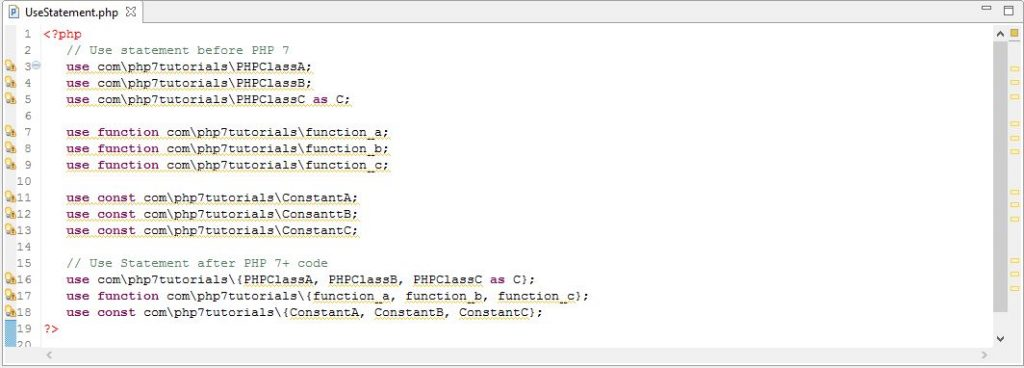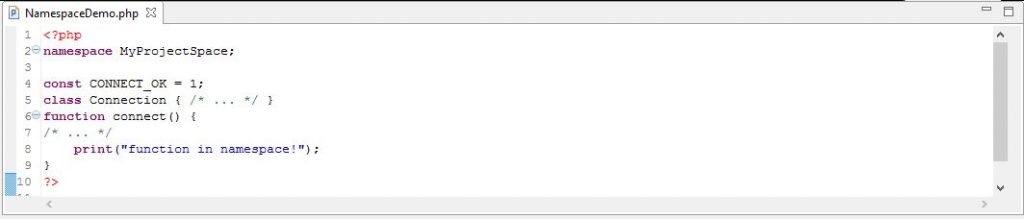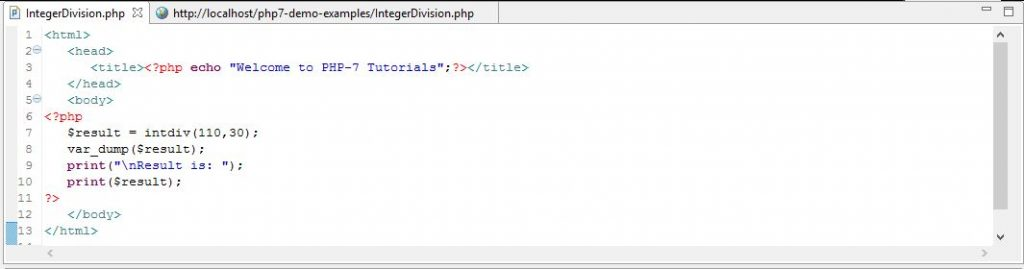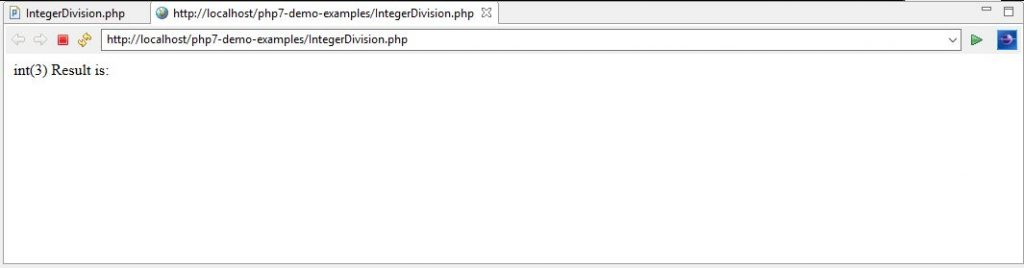Web Programming TutorialsLearn How to Use Statement and Integer Division in PHP 7

# Learn How to Use Statement and Integer Division in PHP 7In the last chapter, we discussed about the new PHP 7 Error handling methodology along with examples. In this chapter, we are going to discuss the ‘use’ statement, which is a new feature and the new Integer division function which are added to PHP 7.

‘Use’ Statement
In PHP 5.x, if we want to import Classes, functions and constants from the same namespace then we have to use the ‘use’ statement multiple times to import each of these elements. However, inPHP7, we can import Classes, functions and constants from the same namespace by using the ‘use’ statement only once that will import all the classes or constants or functions from the same namespace instead of using ‘use’ statements multiple times as in the case of PHP 5.x versions. Thus, PHP 7 has reduced the number of lines of that are occupied by the ‘use’ statement as shown below. Here, we are grouping all classes or functions or constants together that belongs to the same namespace within the curly braces where each of these elements are separated by comma.Purpose of use keyword
In PHP, we can accomplish aliasing with the ‘use’ statement. The main purpose of use keyword is to help PHP compiler to decide which class to execute. Consider, we have two classes with the same name, this could happen when we are working with huge MVC structure application. If we have two classes with the same name, then put them in different namespace. Now suppose that our autoloader is loading both classes and we want to use object of such a class then the compiler will get confused which class to load as both of these classes are with same name. In such a scenario we can use ‘use’ statement to help the compiler in making a decision on what class to load among the two same name classes for the current execution.

Namespace

• Any valid PHP code can be contained within a namespace. If such PHP code types are for classes, interfaces, functions and constant then these types of code are affected by namespace.
• Namespace are always declared by using the namespace It should be noted that, if we declare namespace in a file, then it should always be at the top in the PHP file i.e. before any PHP code other than declare keyword.
• Even no non-PHP code may precede a namespace declaration this includes extra whitespace as well.
• We can define the same namespace in multiple files. This allows the splitting of a namespace’s content across the filesystem.
• The important feature of namespaces is the ability to refer to an external fully qualified name with an alias which is also known as importing. It is equivalent to the ability of the UNIX-based filesystems to create symbolic links to a directory or to a file.
• Three kinds of aliasing or importing are supported by all PHP versions. They are aliasing a class name, aliasing an interface name, and aliasing a namespace name. However, PHP 5.6+ and PHP 7 versions allows aliasing or importing function and constant names.
• This is to be noted that the ‘use’ keyword must be used in the global scope or inside the namespace declarations. The global scope is required because importing is done at the compile time and not at the runtime. Hence, it cannot be blocked or limited to the local scope.
• Following is the example where namespace is used in the PHP code.Explanation of PHP Code

• This is a simple example of namespace used in the PHP code.
• As explained earlier, the namespace is used at the top of the PHP file.
• Next, we are declaring constant, class and function in the same file.
• We can import these PHP code elements in the other PHP file with the help of ‘use’ statement as explained earlier.

Integer Division
In PHP 7, a new function is introduced that performs the integer division on the values passed as operands and returns the division result as int i.e. the integer part only. The syntax of this function is shown below.

intdiv (arg1, arg2)
Here, the first parameter is the numerator and the second parameter is the divisor. Following is the example that demonstrates its operation in detail.Explanation of the PHP 7 Code

• Here, we have defined a variable ‘\$result’ that take the output of the ‘intdiv (110, 30)’ function (Integer division function). This function accepts two parameters, the first parameter is the numerator that is passed as 110 and the second parameter is the divisor that is passed as 30.
• If we do simple mathematics, then we know the output will be 3.666 (approximately). But since this is the integer division therefore it will return only 3 after omitting all the decimal part of the return value.
• Next, we have used the ‘var_dump ()’ function. This function in PHP is used to simply dump the information about the variable. Therefore, in this case you will observe the data type of ‘\$result’ as int. Since this variable holds the return value from the integer division function which returns the value of Integer type.
• Lastly, we are printing the value present in the ‘\$result’ variable which is nothing but the integer part of the result of division of 110 by 30 that is 3. This will be printed on the screen.

Output
When we execute the above PHP 7 program, we will observe the following output. In the output, we can observe the dumped information about the ‘\$result’ variable which is of type integer and has value as 3. Later we are printing the value of this value of screen.Source Code for Statement and Integer Division
Conclusion
In this chapter, we have discussed about the ‘use’ statement and the operation of the new Integer division function, which are added to PHP 7 as oppose to PHP 5.x. In the coming chapter of this tutorial, we are going to discuss about the session options that is added to PHP7.

### Startup Management Through Link Building: Experiencing Standout Success as Early as Possible

21,501Fans
4,212Followers
104,000Subscribers

### Startup Management Through Link Building: Experiencing Standout Success as Early as Possible

- Advertisement -